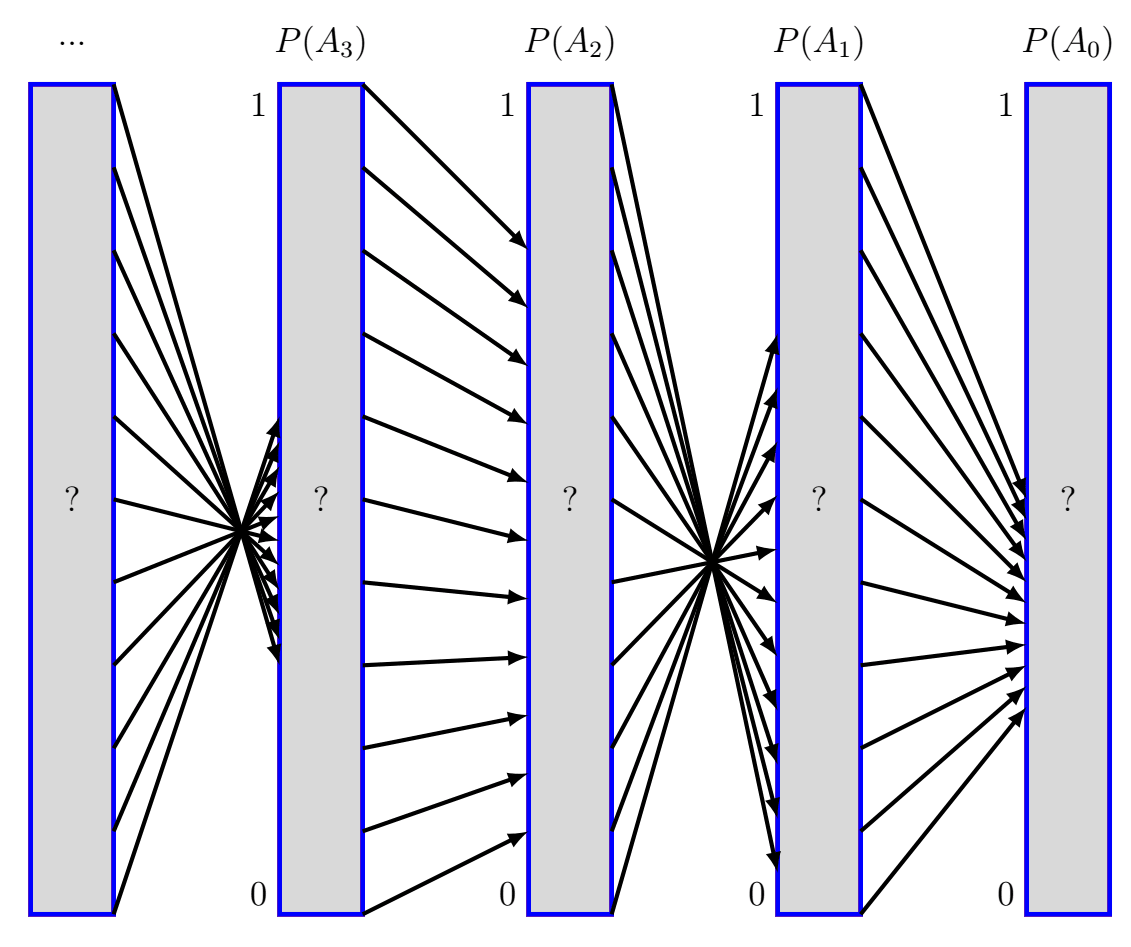# 33

(I thought about this idea on my own before Googling to see if anyone had already written it up. I found something very similar at https://projecteuclid.org/journals/notre-dame-journal-of-formal-logic/volume-50/issue-2/Justification-by-an-Infinity-of-Conditional-Probabilities/10.1215/00294527-2009-005.full so all credit for this line of thinking should go to the authors of that paper. Still, I think that this concept deserves a writeup on Lesswrong and I also want to write a series of posts on this kind of topic so I need to start somewhere. If this idea has already been written up on Lesswrong then please let me know!)

Alice and Bob are driving in a car and Alice wants to know whether the driver in front of them will turn at the next light.

Alice asks Bob, "What's the probability that the driver will turn at the next light?" Unfortunately, Bob doesn't know how to estimate that. However, Bob does know that there are cherry blossoms which might be in bloom off the next exit. Bob is able to use his predictive talent to determine that there's a 50% chance that the driver will turn if there are cherry blossoms on display and that there's a 25% chance that the driver will turn if there aren't any cherry blossoms on display. Bob tells Alice that no other variables will interfere with these conditional probabilities.

Alice then asks Bob, "What's the probability that there will be cherry blossoms on display?" Again, Bob is unable to determine this probability. However, Bob does know that the city government was considering chopping the cherry trees down. Bob tells Alice that if the city chopped them down then there's a 5% chance of finding cherry blossoms and that if the city didn't chop them down then there's a 70% of finding cherry blossoms. Bob knows that no other variables can impact these conditional probabilities.

Alice now asks Bob, "What's the probability that the city cut down the cherry trees?" Predictably, Bob doesn't know how to answer that. However, Bob again uses his magical powers of perception to deduce that there's an 80% chance the city chopped them down if the construction company that was lobbying for them to be cut down won its appeal and a 10% chance the city chopped them down if the construction company that was lobbying for them to be cut down lost its appeal.

Now imagine that this conversation goes on forever: whether the construction company won is determined by whether the pro-business judge was installed which is determined by whether the governor was under pressure and so on. At the end we get an infinite Bayesian network that's a single chain extending infinitely far in one direction. Importantly, there's no "starting" node we can assign an outright probability to.

So Alice will never be able to get an answer, right? If there's no "starting" node we have an outright probability for then how can Alice hope to propagate forward to determine the probability that the driver will turn at the light?

I claim that Alice can actually do pretty well. Let's draw a picture to see why:I'm using  to denote the event where the driver turns right,  to denote the event where the cherry blossoms are on display, and so on. If we know  for positive integer  then we can compute  via

where  and  are the constants which Bob has provided to Alice. Let's think of these as functions  defined by  where we know that . I've illustrated the behavior of these functions with black arrows in the diagram above.

Alice wants to find . What can she do? Well, she knows that  must be an output of , i.e. . Visually:

Alice also knows that  is an output of , so actually :

Alice can keep going in this manner, starting farther and farther back to get narrower and narrower bounds on . Ultimately, Alice knows that:

It would be really nice if this intersection contained only a single element, i.e. our chain of conditional probabilities actually specified a value of . When does that happen? Well,  will shrink any input interval by a factor of , so if Alice wants to actually get a single answer for  then she needs , or equivalently

That's actually a very reasonable condition! For example, if  and  are constant across  and aren't exactly a distance of  apart (i.e. one is  and the other is ) then our condition is satisfied. Therefore, if we're presented with an infinitely long descending chain of conditional probabilities then we should expect the non-conditional probabilities along the chain to be uniquely determined.

1. ^

Hmmm, Bob seems really good at computing these conditional probabilities, and these events pretty reliably seem to only ever depend on one additional variable. I want to relax some of these assumptions in future posts.

2. ^

I'm going to be very mathematically sloppy in this post. I think it's important to ask what our probability space actually is in this setting: there are uncountably infinitely many outcomes (i.e. assignments of values to variables) so we can no longer assume that our probability measure will be induced by a probability mass function. If I were being more formal I would want to check that we can construct probability spaces with these conditional probabilities like we think we can.

3. ^

Assuming we don't have  for any positive .

New Comment